# Fraction calculator

The calculator performs basic and advanced operations with fractions, expressions with fractions combined with integers, decimals, and mixed numbers. It also shows detailed step-by-step information about the fraction calculation procedure. Solve problems with two, three, or more fractions and numbers in one expression.

## Result:

### 3/2 + 1/3 = 11/6 = 1 5/6 ≅ 1.8333333

Spelled result in words is eleven sixths (or one and five sixths).

### How do you solve fractions step by step?

1. Add: 3/2 + 1/3 = 3 · 3/2 · 3 + 1 · 2/3 · 2 = 9/6 + 2/6 = 9 + 2/6 = 11/6
For adding, subtracting, and comparing fractions, it is suitable to adjust both fractions to a common (equal, identical) denominator. The common denominator you can calculate as the least common multiple of both denominators - LCM(2, 3) = 6. In practice, it is enough to find the common denominator (not necessarily the lowest) by multiplying the denominators: 2 × 3 = 6. In the following intermediate step, the fraction result cannot be further simplified by canceling.
In other words - three halfs plus one third = eleven sixths.

#### Rules for expressions with fractions:

Fractions - use the slash “/” between the numerator and denominator, i.e., for five-hundredths, enter 5/100. If you are using mixed numbers, be sure to leave a single space between the whole and fraction part.
The slash separates the numerator (number above a fraction line) and denominator (number below).

Mixed numerals (mixed fractions or mixed numbers) write as non-zero integer separated by one space and fraction i.e., 1 2/3 (having the same sign). An example of a negative mixed fraction: -5 1/2.
Because slash is both signs for fraction line and division, we recommended use colon (:) as the operator of division fractions i.e., 1/2 : 3.

Decimals (decimal numbers) enter with a decimal point . and they are automatically converted to fractions - i.e. 1.45.

The colon : and slash / is the symbol of division. Can be used to divide mixed numbers 1 2/3 : 4 3/8 or can be used for write complex fractions i.e. 1/2 : 1/3.
An asterisk * or × is the symbol for multiplication.
Plus + is addition, minus sign - is subtraction and ()[] is mathematical parentheses.
The exponentiation/power symbol is ^ - for example: (7/8-4/5)^2 = (7/8-4/5)2

#### Examples:

subtracting fractions: 2/3 - 1/2
multiplying fractions: 7/8 * 3/9
dividing Fractions: 1/2 : 3/4
exponentiation of fraction: 3/5^3
fractional exponents: 16 ^ 1/2
adding fractions and mixed numbers: 8/5 + 6 2/7
dividing integer and fraction: 5 ÷ 1/2
complex fractions: 5/8 : 2 2/3
decimal to fraction: 0.625
Fraction to Decimal: 1/4
Fraction to Percent: 1/8 %
comparing fractions: 1/4 2/3
multiplying a fraction by a whole number: 6 * 3/4
square root of a fraction: sqrt(1/16)
reducing or simplifying the fraction (simplification) - dividing the numerator and denominator of a fraction by the same non-zero number - equivalent fraction: 4/22
expression with brackets: 1/3 * (1/2 - 3 3/8)
compound fraction: 3/4 of 5/7
fractions multiple: 2/3 of 3/5
divide to find the quotient: 3/5 ÷ 2/3

The calculator follows well-known rules for order of operations. The most common mnemonics for remembering this order of operations are:
PEMDAS - Parentheses, Exponents, Multiplication, Division, Addition, Subtraction.
BEDMAS - Brackets, Exponents, Division, Multiplication, Addition, Subtraction
BODMAS - Brackets, Of or Order, Division, Multiplication, Addition, Subtraction.
GEMDAS - Grouping Symbols - brackets (){}, Exponents, Multiplication, Division, Addition, Subtraction.
Be careful, always do multiplication and division before addition and subtraction. Some operators (+ and -) and (* and /) has the same priority and then must evaluate from left to right.

## Fractions in word problems:

• Kelly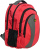Kelly and Dan are collecting clothes for a clothing drive. Dan collected 1/2 as many clothes as Kelly did. If Kelly collected 3 bags of clothes, how many bags of clothes did Dan collect?
• Paper clipsMrs. Bright is organizing her office supplies. There are 5 open boxes of paper clips in her desk drawer. Each box has 1/2 of the paper clips remaining. How many boxes of paper clips are left?
• Samuel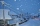Samuel has 1/3 of a bag of rice and Isabella has a 1/2 bag of rice. What fraction of are bag of rice do they have altogether?
• Juan is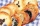Juan is making cookies. He makes 2 batches on Monday and 4 batches on Tuesday. He uses 3/4 cup of flour in each batch. How much flour does juan use?
• Obtuse angleWhich obtuse angle is creating clocks at 17:00?
• Unit rateFind unit rate: 6,840 customers in 45 days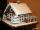Janka and Marienka calculated that there are 210 gingerbreads on the gingerbread house. Janko ate one-seventh of all gingerbreads, and Marienka ate a third less than Janko. How many gingerbreads remained in the gingerbread house?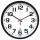How many minutes is a third of an hour? Do you know to determine a third of the lesson hour (45min)?Grandmother baked cakes. Grandfather ate half, then quarter of the rest ate Peter and Paul ate half of rest. For parents left 6 cakes. How many cakes maked the grandmother?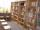The school library contains 6300 books. With this constitutes 7% of professional books for teachers 18% interest books and encyclopedias for pupils and the rest of the fairy tales. How much are which books?Solve inequality: 3x + 6 > 14A sum of money is shared between Peter, John and Henry in the ratio 2:3:5. a) express Henry's share as fraction of John's share. b) what fraction of the whole sum of money is John's share?A recipe requires 3/4 cups of milk. Paula is making 1/2 of the recipe. How many cups will Paula use?